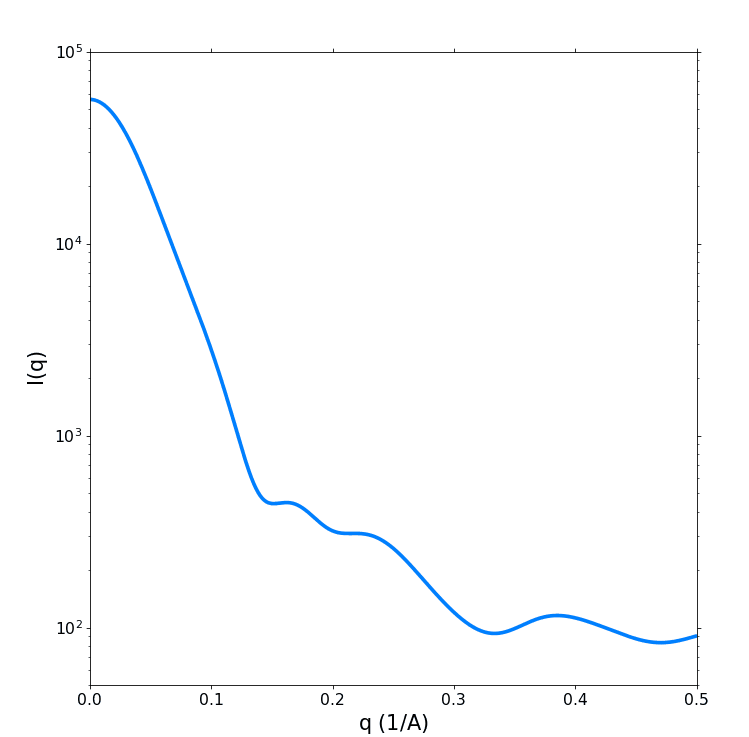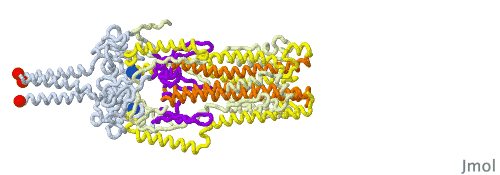## Analytical Calculation of the Scattering Curve I(q) and the Real Space Function p(r) of a Simple Model (Example).Their (identical) scattering curve (left: log(I) vs q) and real space function (right: p(r) vs r) are displayed below:Author: M.Kriechbaum, TU-Graz (2021), e-mail: manfred.kriechbaum@tugraz.at